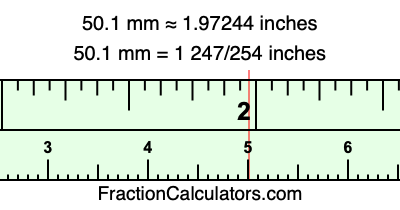50.1 mm in inchesHere is how to convert 50.1 mm to inches. We will give you the fractional answer, the decimal answer, and illustrate the answer on our tape measure.

One millimeter is equal to 5/127 inches, and 50.1 as a fraction is 501/10. Therefore, to get the fractional answer to 50.1 mm in inches, we multiply 501/10 by 5/127 and simplify if necessary.

cm × 5/127 = inches
501/10 × 5/127 = inches
501/10 × 5/127 = 1 247/254
501/10 mm = 1 247/254 inches
50.1 mm = 1 247/254 inches

As promised above, we also have the answer to 50.1 mm in inches in decimal form. Although the fractional answer above is exact, the decimal answer may be rounded if necessary:

1 247/254 ≈ 1.97244094488189
1 247/254 ≈ 1.97244
50.1 mm ≈ 1.97244 inches

Our image below shows 50.1 mm on a tape measure. The top row of the tape measure is inches, and each mark on the bottom row is 1 millimeter (10 millimeter marks equal 1 centimeter).As you can see, we drew a red line where 50.1 mm and 1.97244 inches meet on the measuring tape.

Millimeters to Inches Calculator
Here you can convert another length in millimeters to inches.

Convert  mm to inches.

What is 50.2 mm in inches?
Here is another inches to millimeters calculation that we have done for you.